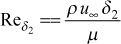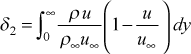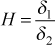# Institut für Thermodynamik der Luft- und Raumfahrt - Universität StuttgartMechanical Engineering - The University of Texas at Austin# Reynolds number

x-Reynolds Number - External boundary layer flows use different Reynolds numbers. The most common is the x-Reynolds number, a carryover from isothermal aerodynamics theory. It is formulated using the idea of a virtual origin,The use of a virtual origin is not particularly useful for laminar flows of the Falkner-Skan type when m=0 (Blasius, zero pressure gradient flow) or for the m=1 (Hiemenz or two-dimensional stagnation point flow, and the approximation for the cylinder in crossflow or leading edge of the gas turbine blade). Its main use is in controlling the starting value of the momentum thickness Reynolds number for turbulent boundary layer flow and kstart=3.

Momentum Thickness Reynolds Number - The momentum thickness Reynolds number is given bywhere the momentum thickness for planar flows is calculated by evaluating the integralDisplacement Thickness - The corresponding displacement thickness for planar flow is evaluated from the integral.

Shape Factor - The classic shape factor represents the ratio of these two integralsEnthalpy Thickness Reynolds Number - For heat transfer there is an enthalpy thickness and corresponding Reynolds number that is customarily used in heat transfer correlations. The enthalpy thickness Reynolds number is given bywhere the enthalpy thickness for planar flows is calculated by evaluating the integralThese integrals are computed with variable properties if kfluid>1. Note that TEXSTAN has corrections to these integrals for thick axisymmetric flows (kgeom=2,3) but they rarely make a difference. This idea is briefly discussed in Hokenson1.

## References

1
Hokenson, G. J., (1977 "Consistent Integral Thickness Utilization for Boundary Layers with Transverse Curvature," AIAA Journal, Vol. 15, pp. 597-600.

website updated Sept 2010   © 1996-2010 Michael E. Crawford - all rights reserved - website validated for CSS 2.1 and XHTML 1.0 strict at www.w3.org